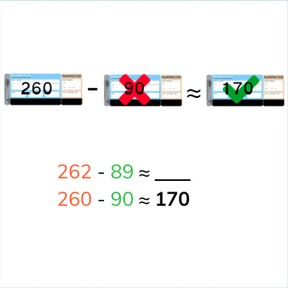Estimate the difference to 1,000

# Estimate the difference to 1,000

No account needed.8,000 schools use Gynzy92,000 teachers use Gynzy1,600,000 students use Gynzy

## General

Students learn to estimate the difference with numbers to 1,000 and learn to round numbers to the nearest ten, or hundred.

3.NBT.A.2

## Relevance

Discuss with students that it is important to learn to estimate the difference so you can quickly determine about how much you have left. An example is if you buy tickets and want to know about how much money you have left.

## Introduction

Start the lesson by practicing rounding numbers. Have students look at the number line and determine which number is closest. Drag the numbers to their nearest hundred. Then practice rounding to a tens number or rounding within the hundreds number.

## Development

Explain to students that when estimating the difference you start by rounding the numbers and then subtracting them. The problem of 489 - 197 is rounded to 500 - 200. Explain that doing so makes the subtraction problem easier. Show students that they sometimes round to a hundreds number, and sometimes to a tens number within the hundred. If you round to the nearest hundred, it is easier, but if you round to the nearest ten within the hundred, your estimation will be more precise. Show the differences between both types of rounding and show example problems using both. Have students practice matching subtraction problems to their rounded counterparts. Explain that you can also have estimation subtraction problems with multiple subtrahends. You can choose to take them away one by one after rounding them, or you can add them together and take away both in one step. Practice with rounding in a real life context. Ask students to estimate about how many students are left when on a field trip, and then ask about how much money they'll have left after buying a laptop. Practice rounding as precisely as possible to get an accurate estimate.

Check that students are able to estimate the difference with numbers to 1,000 by asking the following questions:
- Why is it useful to be able to round numbers and estimate subtraction?
- How do you round a number? How do you round 389 or 573?
- When do you round to a ten and when do you round to a hundred?
- If you want to estimate as quickly as possible, what should you round to?
- If you want to estimate as precisely as possible, what should you round to?

## Guided practice

Students are given abstract and story problems in which they must estimate the difference. Students are asked to fill in the blank or select from the correct answer given.

## Closing

Discuss with students that it is useful to be able to estimate the difference so you can easily determine about how much you have left. Discuss why you might round to a ten or a hundred, what is the difference? When you round more exactly, your answer is also more exact. To close ask students to challenge each other. Each student must write three subtraction problems with numbers to 1,000 in their notebooks. Then they must write the rounded subtraction problems in random order, and then they must write the estimated differences. They trade notebooks with a classmate and must link the original subtraction problem, the rounded subtraction problem, and the difference. Then as a class practice a few estimation problems to check that students are comfortable estimating the difference with numbers to 1,000.

## Teaching tips

Students who have difficulty with this learning goal can be supported by practicing rounding to hundreds numbers. When they are comfortable with solving subtraction problems rounded to hundreds numbers, they can practice rounding to the tens within the hundreds number.

### The online teaching platform for interactive whiteboards and displays in schools

• Save time building lessons

• Manage the classroom more efficiently

• Increase student engagement